Slow Manifold Gallery / Flow Manifold Gallery

Singularly Perturbed Systems 2D & 3D

MF 30 Van der Pol model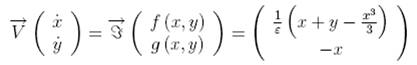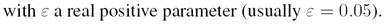Flow Curvature Method, i.e., the 1st flow curvature manifold directly provides the slow invariant manifold associated with Van der Pol model.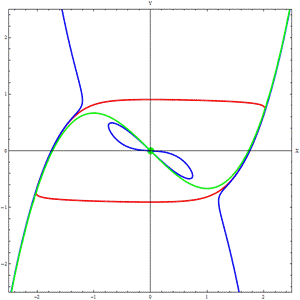1st flow curvature manifold

singular approximation

 Van der Pol, B., “On ’Relaxation-Oscillations’,” Phil. Mag., 7, Vol. 2.

MF 48 FitzHugh-Nagumo model

The FitzHugh-Nagumo model (FitzHugh, 1961 ; Nagumo et al., 1962) is a simplified version of the Hodgkin-Huxley model which models in a detailed manner

activation and deactivation dynamics of a spiking neuron. In the original papers of FitzHugh this model was called “Bonhoeffer-Van der Pol oscillator”, since it

contains the van der Pol oscillator as a special case for a = b = 0.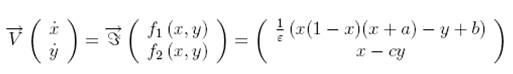x denotes the membrane potential, y is a recovery variable and b is the magnitude of stimulus current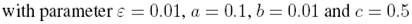Flow Curvature Method, i.e., the 1st flow curvature manifold directly provides the slow invariant manifold associated with FitzHugh-Nagumo model.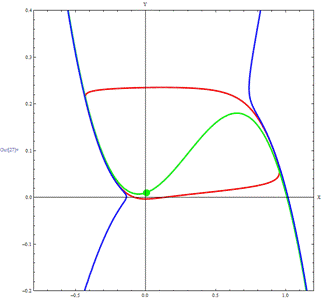1st flow curvature manifold

singular approximation

 FitzHugh, R., “Impulses and physiological states in theoretical models of nerve membranes,” 1182-Biophys. J., 1, 445-466.

 Nagumo J. S., Arimoto S. & Oshizawa S., “An active pulse transmission line simulating nerve axon,” Proc. Inst. Radio Engineers. 50, 2061-2070.

MF49 cubic Chua’s model

The cubic Chua’s circuit (Chua et al., 1986) is an electronic circuit comprising an inductance L1, an active resistor R, two capacitors C1 and C2, and a nonlinear

resistor. Chua’s circuit can be accurately modeled by means of a system of three coupled first-order ordinary differential equations in the variables x (t), y (t) and

z (t), which give the voltages in the capacitors C1 and C2, and the intensity of the electrical current in the inductance L1, respectively.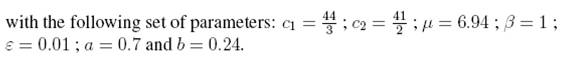The function k(x) describes the electrical response of the nonlinear resistor, i.e., its characteristics which is a “cubic” function is defined by: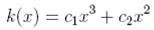where the real parameters c1 and c2 are determined by the particular values of the circuit components.

Flow Curvature Method, i.e., the 2nd flow curvature manifold directly provides the slow invariant manifold associated with cubic Chua’s model.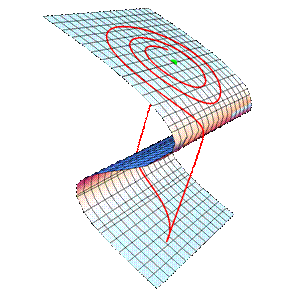Chua, L. O., Komuro, M. & Matsumoto, T., “The double scroll family,” IEEE Trans. on Circuits and Systems, 33, Vol.11, 1072-1097.

Non-singularly Perturbed Systems 2D & 3D

MF 50 Brusselator model

Studying an hypothetical chemical reaction in which it is assumed that the reactions are all irreversible a Belgium group (Prigogine et al., 1967) proposed the following model in which x and y denotes concentrations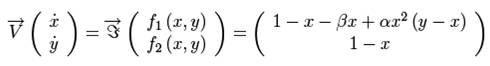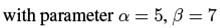According to Grasman (1987) the chemical oscillation turns into relaxation oscillations. Thus, the Brusselator may be considered as slow-fast dynamical systems although it has no small multiplicative parameter in its velocity vector field and so no singular approximation.

Although the Brusselator model is not singularly perturbed it can be considered as a slow fast dynamical system since it may be shown (numerically) that its functional Jacobian matrix exhibits a fast eigenvalue.

Flow Curvature Method, i.e., the 1st flow curvature manifold directly provides the slow invariant manifold associated with Brusselator model.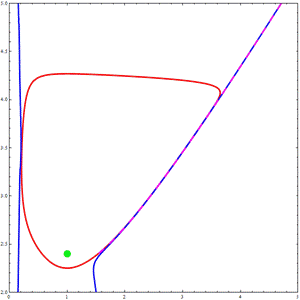Prigogine, I. & Lefever, R. “On Symmetry-Breaking Instabilities in Dissipative Systems,” Journal of Chemical Physics, Vol. 46, 3542-3550.

 Grasman, J., Asymptotic Methods for Relaxation Oscillations and Applications, vol. 63, Springer-Verlag, New York.

MF 50 Lorenz model

In the beginning of the sixties a young meteorologist of the M.I.T., Edward N. Lorenz, was working on weather prediction. He elaborated a model derived from

the Navier-Stokes equations with the Boussinesq approximation, which described the atmospheric convection. Although his model lost the correspondence to the actual atmosphere in the process of approximation, chaos appeared from the equation describing the dynamics of the nature. Let’s consider the Lorenz model (Lorenz, 1963):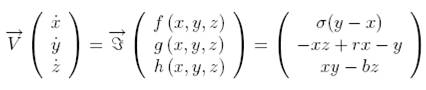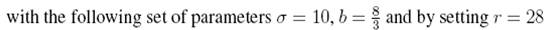Although the Lorenz model is not singularly perturbed it can be considered as a slow fast dynamical system

since it may be shown (numerically) that its functional Jacobian matrix exhibits a fast eigenvalue.

Flow Curvature Method, i.e., the 2nd flow curvature manifold directly provides the slow invariant manifold associated with Lorenz model.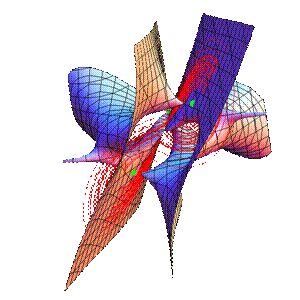Lorenz, E. N., “Deterministic non-periodic flows,” J. Atmos. Sc, 20, 130-141.

MF 51 Pikovskii-Rabinovich-Trakhtengerts model

The Pikovskii-Rabinovich-Trakhtengerts model (PRT) (Pikovskii et al., 1978) has been elaborated in order to study interactions between “whistler waves” which propagate parallel to the magnetic field and lower hybrid waves in a plasma. Such interactions are among the important phenomena taking place in the ionosphere. This phenomenon can be modeled by means of a system of three coupled first-order ordinary differential equations in the variables x(t), y(t) and z(t), which give the the normal amplitude of the wave, the normal amplitude of the ion acoustic wave and the normal amplitude of the synchronous third wave produced, respectively. The amplitudes are assumed to be constant in space. The evolution equations of the (PRT) model may be written in dimensionless form: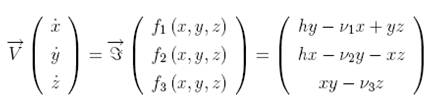where the amplitudes have been nondimensionalized; h is proportional to the amplitude of the electric field of the “whistler” and v1 and v2 are the damping decrements of the excited hybrid and acoustic waves normalized to the damping of the decay-induced third wave.

It has been recently established (Llibre et al., 2008) that this dynamical system exhibits a four wings butterfly chaotic attractor for a particular set of parameters.

Although the (PRT) model is not singularly perturbed it can be considered as a slow fast dynamical system

since it may be shown (numerically) that its functional Jacobian matrix exhibits a fast eigenvalue.

Flow Curvature Method, i.e., the 2nd flow curvature manifold directly provides the slow invariant manifold associated with (PRT) model.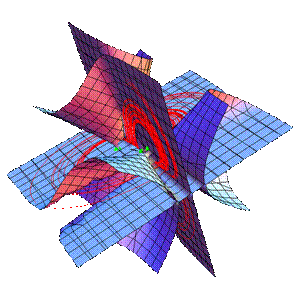Pikovskii, A. S., Rabinovich, M. I. & Takhtengerts, V. Y., “Onset of stochasticity in decay confinement of parametric instability,” Soc. Phys. J. E. T. P., t. 47, 715-719.

 Llibre, J., Messias, M. & Silva, P. R., “On the global dynamics of the Rabinovich system,” J. Phys. A: Math. Theor. 41, 275210.

Singularly Perturbed Systems 4D & 5D

MF 55 fourth-order cubic Chua’s model

The fourth-order cubic Chua’s circuit (Thamilmaran et al., 2004, Liu et al., 2007) may be described starting from the same set of differential equations as previously but while replacing the piecewise linear function by a smooth cubic nonlinear.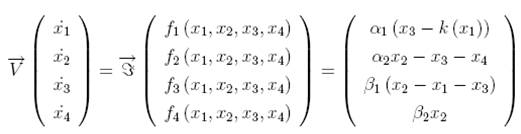The function k (x1) describing the electrical response of the nonlinear resistor is an odd-symmetric function similar to the piecewise linear nonlinearity k (x1) for which the parameters c1 = 0.3937 and c2 = -0.7235 are determined while using least-square method (Tsuneda, 2005) and which characteristics is defined by: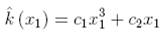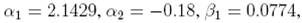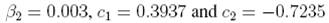Flow Curvature Method, i.e., the 3rd flow curvature manifold directly provides the slow invariant manifold associated with cubic Chua’s model.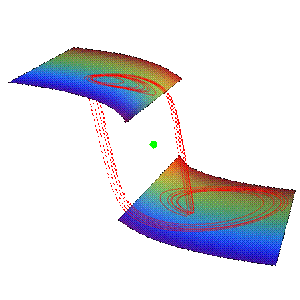Thamilmaran, K., Lakshmanan M. & Venkatesan A. “Hyperchaos in. a modified canonical Chua’s circuit,” Int. J. Bifurcation and Chaos, vol. 14, 221-243.

 Liu X., Wang, J. & Huang, L., “Attractors of Fourth-Order Chua’s Circuit and Chaos Control,” Int. J. Bifurcation and Chaos 8, Vol. 17, 2705 -2722.

MF 56 fifth-order cubic Chua’s model

The fifth-order cubic Chua’s circuit (Hao et al., 2005) may be described starting from the same set of differential equations as previously but while replacing the

piecewise linear function by a smooth cubic nonlinear.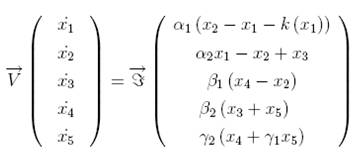The function k (x1) describing the electrical response of the nonlinear resistor is an odd-symmetric function similar to the piecewise linear nonlinearity k (x1) for which the parameters c1 = 0.1068 and c2 = -0.3056 are determined while using least-square method (Tsuneda, 2005) and which characteristics is defined by: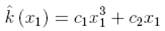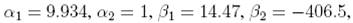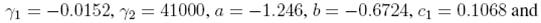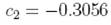Flow Curvature Method, i.e., the 4th flow curvature manifold directly provides the slow invariant manifold associated with cubic Chua’s model.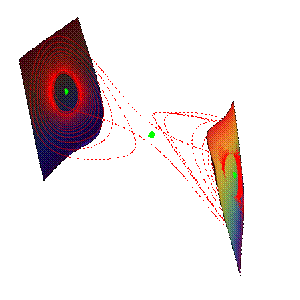Hao, L., Liu, J. & Wang, R., “Analysis of a Fifth-Order Hyperchaotic Circuit,” in IEEE Int. Workshop VLSI Design & Video Tech., 268-271.

 Tsuneda, A., “A gallery of attractors from smooth Chua’s equation,” Int. J. Bifurcation and Chaos, vol. 15, 1- 49.

Non-singularly Perturbed Systems 4D & 5D

MF 57 Homopolar dynamo model

Homopolar dynamo model are used for the understanding of spontaneous magnetic field generation in magnetohydrodynamics. In the seventies H. K. Mofatt (1978) proposed a model of coupled Faraday-disk homopolar dynamos. By introducing fluxes variables instead of the currents this model has been transformed (Hide et al., 1999) into the following dimensionless one: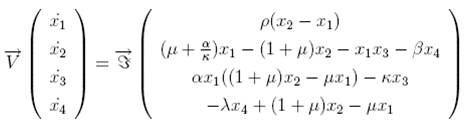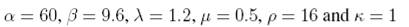Although the homopolar dynamo model is not singularly perturbed it can be considered as a slow fast dynamical system since it may be shown (numerically) that its functional Jacobian matrix exhibits a fast eigenvalue.

Flow Curvature Method, i.e., the 3rd flow curvature manifold directly provides the slow invariant manifold associated with Mofatt model.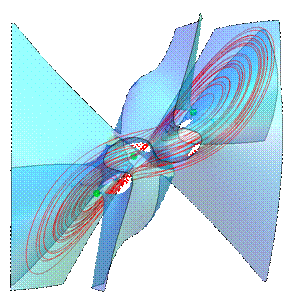Mofatt, H. K., Magnetic field generation in electrically conducting fluids, Monograph in CUP series on Mechanics and Applied Mathematics. (Translated from Russian).

 Hide, R. & Moroz, I. M., “Effects due to induced azimuthal eddy currents in a self-exciting Faraday disk homopolar dynamo with a nonlinear series motor I. Two special cases,” Phys. D, Vol. 134, 2, 287-301.

MF 58 Magnetoconvection model

A fifth-order system for magnetoconvection (Knobloch et al., 1981) is designed to describe nonlinear coupling between Rayleigh-Bernard convection and an external magnetic field. This type of system was first presented by Veronis (Veronis, 1966) in studying a rotating fluid. The fifth-order system of magnetoconvection is a straightforward extension of the Lorenz model for the Boussinesq convection interacting with the magnetic field. The fifth-order autonomous system of magnetoconvection is given as follows: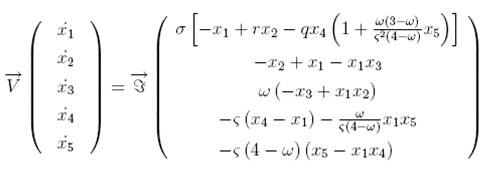where x1(t) represents the first-order velocity perturbation, while x2 (t), x3 (t), x4 (t) and x5 (t) are measures of the first- and the second-order perturbations to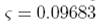the temperature and to the magnetic flux function, respectively. With the five real parameters where                          is the magnetic Prandtl number (the ratio of the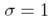magnetic to the thermal diffusivity),           is the Prandtl number, r = 14.47 is a normalized Rayleigh number, q = 5 is a normalized Chandrasekhar number, and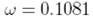is a geometrical parameter

Although the magnetoconvection model is not singularly perturbed it can be considered as a slow fast dynamical system since it may be shown (numerically) that its functional Jacobian matrix exhibits a fast eigenvalue.

Flow Curvature Method, i.e., the 4th flow curvature manifold directly provides the slow invariant manifold associated with magnetoconvection model.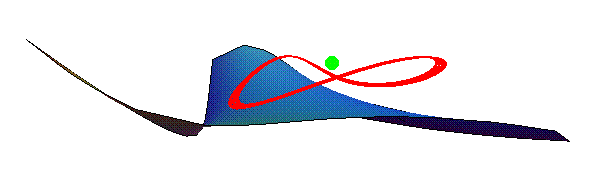Veronis, G., “Motions at subcritical values of the Rayleigh number in a rotating fluid,” J. Fluid Mech., Vol. 24, 545–554.

 Knobloch, E. & Proctor, M., “Nonlinear periodic convection in double-diffusive systems,” J. Fluid Mech. 108, 291-316.

Non-Autonomous Dynamical Systems

MF 60 Forced Van der Pol model

In order to highlight the efficiency of the Flow Curvature Method, i.e., that it may extended to non-autonomous dynamical systems let’s consider the forced Van der Pol model (Guckenheimer et al., 2002) which may be written as: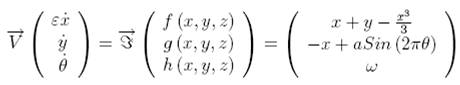A variable changes may transform this non-automous system into an autonomous one which reads: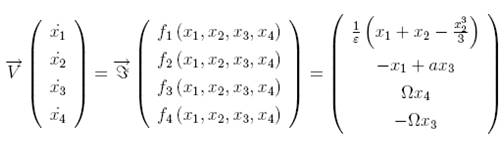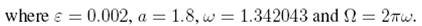Although this transformation increases the dimension of the system the

Flow Curvature Method enables to directly compute the slow manifold analytical equation associated with system.

Flow Curvature Method, i.e., the 3rd flow curvature manifold directly provides the slow invariant manifold associated with Forced Van der Pol model.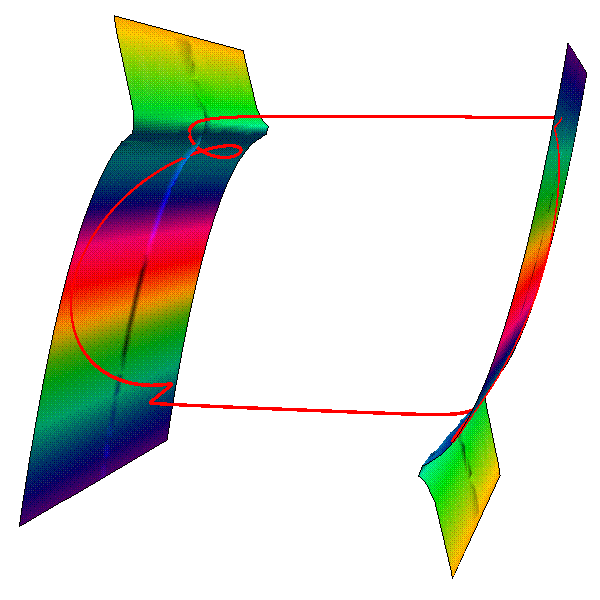Guckenheimer, J., Hoffman, K. & Weckesser, W., “The forced van der Pol equation I: the slow flow and its bifurcations,” SIAM J. App. Dyn. Sys., 2, 1-35.